Inside the Sachs Elan Hub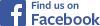Tweet Follow @sheldonbrowncom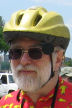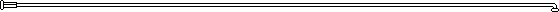There are also articles on this site more generally about internal-gear hubs
and about the history of the Sachs Elan 12-speed hub

## Why Pay Any Attention to This Hub?

I got interested in the Sachs Elan hub as part of my project to revise and expand Sheldon Brown's online Internal-Gear Calculator.

I decided to recalculate the gear ratios of bicycle hubs from first principles -- starting with gear-tooth counts. Manufacturers' published ratios for many hubs have only two places to the right of the decimal point, and are too imprecise to satisfy me: gearing calculations could easily be off by 1% or more in the lower gears. Also, once I'd done the math, a few published ratios proved to be incorrect.

I prepared a Microsoft Excel workbook of the calculations and published the results in a set of Web pages. I gratefully acknowledge the help of other cyclists who sent in tooth counts for hubs which I did not have.

I had some fun with math, too. Unlike measurements, the drive ratios of geared hubs are exact. Some proved to be ratios of rather large numbers. That is to say, you could ride for miles before the gears all came around exactly to where they were at the start.

When I'd finished the calculations for most hubs, one major challenge still loomed over the project: the Sachs Elan 12-speed hub. This hub was only manufactured for a few years. It also had a bad reputation for breakdowns. Still, the Gear Calculator would not be complete without covering this hub.

None of the Elan's drive ratios except the unity ratio fit the same pattern as with other hubs, posing an intriguing mental challenge, and suggesting that there might be something special to learn by studying the Elan.

As described on the main page about the Elan, German cyclist Uno Blau was kind enough to locate an Elan hub and ship it to me. I disassembled it, counted gear teeth, developed the mathematical formula to describe its inner workings, and now I have the results.

I'm pleased to report that the design concept of the Elan was brilliant, and deserves further exploration -- though the execution of the concept was unfortunately flawed. More about that later...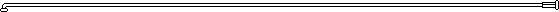## For Gear Freaks...

A warning: from now on, this article gets technical and will appeal mostly to gear freaks -- people who really care about bicycle gearing. I must count myself as a gear freak. To some extent, so was Sheldon Brown. If you are a math head, mechanical or electrical engineer, you also may find the remainder of this article interesting.

You may sign off now, if you like...

...or -- so you didn't. We'll proceed with a description of the Elan's workings.

In the planetary gear systems of most internal-gear bicycle hubs, the sun gear is attached to the axle and does not turn, as described on another page on this site. These systems produce an increase ratio by applying power at the planet gears and taking it off from the ring gear, or vice versa for a decrease ratio. Hubs with more than three speeds use more than one set of sun and planet gears, or use one planetary system after another, so the ratio of the first system is multiplied by that of the second..

The Elan, as it turns out, also places the sun gear in motion, so this hub can perform addition and subtraction, rather than only multiplication. The subtracted amount becomes proportionally smaller as the drive ratios increase, and in this way the Elan achieves narrower ratios toward the top of the range, a very desirable outcome. The table below gives the ratios; it will be repeated later following an explanation of how the Elan achieves them.

Drive ratios of the Sachs Elan Internal-Gear Hub

Step Published
ratio
Calculated
Ratio
Fraction Ratio increment
1 0.697 0.698  203/291
2 0.851 0.852 10987/12901 1.221
3 1.000 1.000 1 1.174
4 1.179 1.179 1512035/1282728 1.179
5 1.333 1.333 3989143/2993032 1.131
6 1.481 1.481 6529/4408 1.111
7 1.612 1.614 258808/160341 1.090
8 1.766 1.768 661518/374129 1.095
9 1.915 1.917 1056/551 1.084
10 2.061 2.061 5287177/2565456 1.075
11 2.217 2.215 13258869/5986064 1.075
12 2.364 2.363 29835/8816 1.067

(A similar approach was used in some mid-20th century Sturmey-Archer hubs to generate ratios very near 1/1, but these hubs had only 3 or 4 speeds and did not take advantage of the narrowing of ratios.)Below is a rough but conceptually accurate half cross-section of the Elan from a Web page posted by German cyclist Stefan Brix. I have enlarged the drawing and added color. The crosshatched and diagonally striped parts in the drawing are axles and frameworks; the parts in solid color are gears. The little rows of dots represent gear teeth.

I have placed a copy of the drawing on another page, so you can continue to view the drawing as you read through the text below. For the greatest convenience, have your Web browser open that page in a separate window. The drawing has labels in German, but I'll translate them, as needed.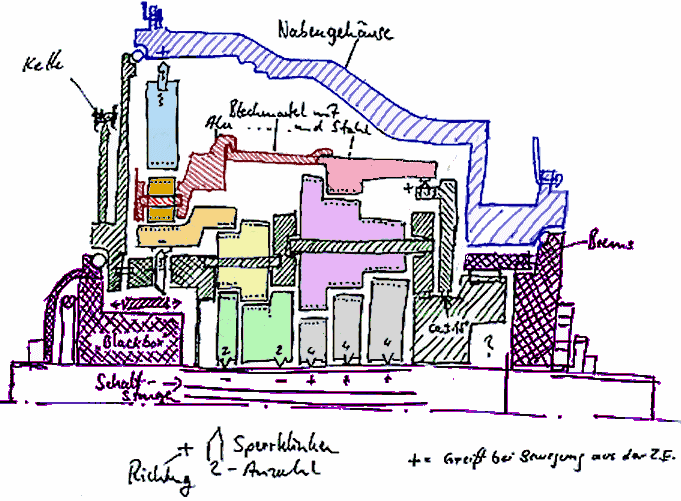## Parts of the Hub

Now, a description of the hub's parts.

• The drawing shows the top half of the hub as seen from the front of the bicycle. The chain ("Kette") and sprocket are at the left in the drawing. The chain pulls toward you. The parts shown in black are connected to the sprocket and turn with it.
• Parts permanently connected to the axle are shown in purple cross-hatching. These parts do not turn. "Brems" at the right is the coaster brake supplied in one version of the hub.
• The hub shell ("Nabengehäuse") is shown in blue striping. This turns at the speed established by the gearing, or it can freewheel.
• The main planetary gear system consists of sun gear a2, planet gears b (one of three is shown) and ring gear c. (These letters are shown in the copy of the drawing on another page.)
• Pawls are the v-shaped, pointy objects in the drawing. A plus sign indicates that a pawl freewheels forward, and vice versa. Some sun gears have two pawls and some have 4, as indicated.
• The framework shown in red striping is driven by an increase gear train (sun gear g, h, or i, compound planet gear j and ring gear k in the drawing on another page.). If the sun gears are all free to turn, the pawls at the right side of gear k turn the framework at the same rate as the sprocket, or else this gear train drives it at one of three higher rates.
• Sun gear d or e, compound planet gear f and ring gear a1 make up a decrease gear train. Gear b turns gear a forward at one of two rates, or else gear a may be held stationary by pawls, one of which is shown just below it.
• Shifting is by means of rods ("Schaltstange") which extend along the axle and which rotate to allow pawls inside sun gears to engage grooves in the axle, or else to glide past. The operation of these rods is controlled by the "black box" shown in the drawing. In addition, the pawl that engages gear a may be engaged or disengaged by the sliding ring shown just above the "black box".
• When gear b goes around the hub axle faster than the sprocket, it drives gear c and the hub shell faster, adding to the speed of the sprocket.
• When gear a goes around the hub axle faster than the sprocket, gear b slows or reverses direction, so gear b drives gear c and the hub shell slower, subtracting from the speed of the sprocket.
• There are 4 x 3 = 12 drive ratios because gear b can go around the hub axle at at the same rate as the sprocket, or three higher rates, and gear a can turn around the hub axle at the same rate as the sprocket, or two higher rates.

All this should be perfectly clear, right? (Well, don't feel bad if it isn't. It took me two days to sort this out. Now, onwards to yet a higher level of geeky-freakiness :-)

## Gear by Gear

Let's look at how the Elan generates its drive ratios.

In the Elan’s lowest ratio, sun gears g, h and i are free to rotate backward, so gear k is driven by the pawls at the right side in the drawing (left side of the hub), turning at the same speed as the sprocket, and carrying planet gears b with it. Gear d is locked to the axle, so gear a goes around the axle faster than the driver. Planet gears b therefore rotate backwards, and so gear ring c and the hub shell turn slower than the driver.

The second-lowest ratio the same as first except that gear e is locked to the axle, resulting in rotation of gear a nearer the speed of the driver and less decrease.

The third gear is direct drive, achieved in an unusual and indirect way.  Sun gear a as well as the axles of planet gears b are locked to the driver. Planet gears b therefore do not turn on their axles, and their teeth are stationary relative to gears a2 and c. Planet gears b transmit power from sun gear a to ring gear c without effecting any change in speed.

The remaining higher drive ratios are achieved like those already described, except that the planetary system of gears i, j and k also carries planet gears b forward. Depending on whether gear j1, j2 or j3 is locked to the axle, the hub achieves drive ratios 4 5 and 6; 7, 8 and 9; or 10, 11 and 12.## Tooth Counts

After disassembling the hub, I came up with the numbers of gear teeth shown in the copy of Stefan Brix's drawing below.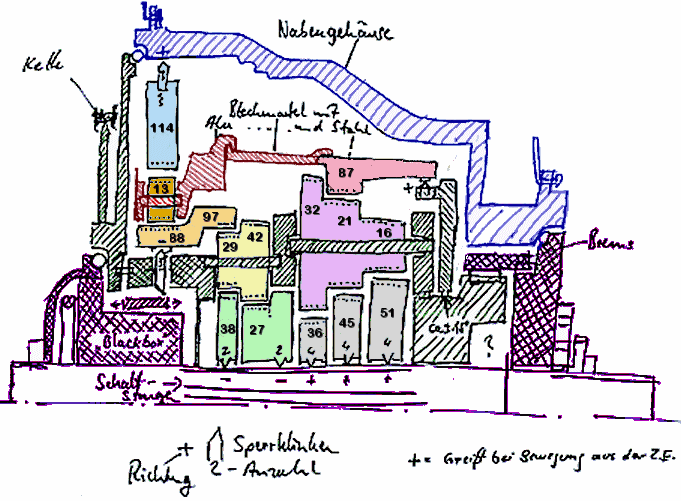The table below gives the same numbers as text for the sake of accessibility and ease of copying.

Main Gear System Decrease Gear System
Increase Gear System
Ring gear 114 97     87
Planet gear 13 29 42 32 21 16
Sun gear 88 38 27 36 45 51

## The Math

This section of the article describes how I calculated the ratios.

Microsoft Excel or other similar computer spreadsheet/workbook software makes it possible to enter numbers directly into a formula; also to duplicate that formula with different input numbers, to generate multiple results all at once. Spreadsheet software is commonly understood as a tool for accountants. It also has widespread applications for scientists and engineers...

The manufacturer's published ratios -- even if inexact -- make it easier to check calculations for the Elan, as for other hubs.

The formulas in the paragraphs below are given in Microsoft Excel format, except that I have inserted the letter names of the Elan's gears when generalizing.

The rotation rate of the Elan's ring gear a1 is:

1. (97 + 38) / 97

times that of the driver.

This rate acts through sun gear a2, with 88 teeth, and planet gears b (tooth count immaterial) to decrease the rotation rate of ring gear c. by

2. (88 * (1 - (97 + 38) / 97)) / 114

However, the entire assembly of planet gears b and ring gear c are rotating forward at the input speed, and so the result is

3. 1 + (88 * (1 - (97 + 38) / 97)) / 114

This gives a result of 0.698, the hub's lowest ratio, which uses the decrease gear train only: the increase gear train and the planet gears of the main gear train are locked to the speed of the driver. The formula may be generalized as

4. 1 + (a2 * (1 - (a1 + d) / a1)) / c

This is the simplest formula for any of the ratios other than the unity ratio.

In the second-lowest gear, with sun gear e, driving gear f1, the formula is

5. 1 + (88 * (1 - (1 + 27 * 29/(97 * 42)))) / 114

This gives a result of .852, and generalizes to

6. 1 + (a2 * (1 - (1 + e * f1 / (a1 * f2)))) / c

Substituting d for e and f1 for f2 in this formula, we get a formula for simple planet gears:

7. 1 + (88 * (1 - (1 + 38 * 29/(97 * 29)))) / 114

which generalizes to

8. c + (a2 * (1 - (1 + d * f1 / (a1 *f1)))) / c

and simplifies to our earlier formula,

9. 1 + (a2 * (1 - (a1 + d) / a1)) / c

So it also works for the lowest gear, and it works for unity ratio, because if the ratio described by

10. (a1 + d) / a1

is 1:1, then the final ratio is c / c, or 1.

When the increase gear train's planet gears are locked to the driver and its middle sun gear is locked to the axle, its ring gear's rate of rotation is

11. (87+45)/87

This is the rate at which the planet gears of the main gear train rotate around the axle.
The differential speed of these planet gears relative to the sun gear is

12. ((87+45)/87) - 1

And so the differential speed imparted to the ring gear relative to the driver is

13. (88 + 114) * (((87+ 45)/87) - 1)/114

and the total speed of the ring gear is

14. 1 + (88 + 114) * (((87 + 45)/87) - 1)/114

This comes out to 1.9165, close to the published ratio of 1.915 for 9th gear, and also works for the 1/1 ratio, as

15. 1 + ((88 + 114)/114) * (1 - 1)

Modifying the formula to account for the compound planetary gears, the ratio of the modifying stage in the hub's highest ratio is

16. 1 + 51 * 21/(87 * 16)

Then the overall formula is

17. 1 + (88 + 114) * ((1 + 51 * 21/(87 * 16)) - 1)/114

Which generalizes to

18. 1 + (a2 + c) * ((1 + i * j2 / (k * j3)) - 1) / c

This is the formula for 12th gear. It also works for the unity ratio when substituting 1 for the term

19. i * j2 / (k * j3)

for 6th when substituting

20. g * j2 / (k * j1)

and for 9th, when substituting

21. h * j2 / (k * j2)

The ratio for the remaining gears (4th, 5th, 7th, 8th, 10th and 11th) which use both modifying gear trains is obtained by adding the decrease formula to the increase formula. The resulting formula works for every drive ratio of the hub. The example below is for the second-highest of the 12 drive ratios:

22. 1 + (88 * (1 - (1 + 27 * 29/(97 * 42))) + (88 +114) * (1 + 51 * 21/(87 * 16) - 1))/114

and it generalizes to:

23. 1 + (a2 * (1 - (1 + e * f1/( a1 * f2))) + (a2 + c) * (1 + i * j2 / (k * j3) - 1)) / c

Substitutions may be made for other ratios, as described above.

The published ratios are below, followed by calculated values also rounded to three places after the decimal point, and then by exact, fractional ratios and by ratio increments. Inaccurate published ratios -- 7 of the 12 -- are shown in red, and the "trick" rounded number is shown in green. Note the smooth decrease in ratio increments from bottom to top of the range.

Drive ratios of the Sachs Elan Internal-Gear Hub

Step Published
ratio
Calculated
Ratio
Fraction Ratio increment
1 0.697 0.698  203/291
2 0.851 0.852 10987/12901 1.221
3 1.000 1.000 1 1.174
4 1.179 1.179 1512035/1282728 1.179
5 1.333 1.333 3989143/2993032 1.131
6 1.481 1.481 6529/4408 1.111
7 1.612 1.614 258808/160341 1.090
8 1.766 1.768 661518/374129 1.095
9 1.915 1.917 1056/551 1.084
10 2.061 2.061 5287177/2565456 1.075
11 2.217 2.215 13258869/5986064 1.075
12 2.364 2.363 20835/8816 1.067## Efficiency

Greater efficiency is achieved by having fewer gear trains under load, and by having them turn more slowly.

The Elan wins on this count in its unity ratio, in which the only gears under load, those of the main system, are not turning in relation to their axles or other gears they engage. The Elan does not do as well in other drive ratios, in which at least two stages are always under load.

In drive ratios 1 and 2, the gears of the decrease system and those of the main stage are turning and carry the full load that is driving the hub shell.

In drive ratios 6, 9 and 12, the gears of the increase system and of the main system are turning and carry the full load that is driving the hub shell.

In the remaining drive ratios, all three systems are under load. The speed of the planet pinions of the final stage is, however, less than it would be if only the increase or decrease system were active.

The increase and decrease systems of the Elan both apply torque to the axle and convert the rotation rate of the driver to a higher one. The decrease system spins its ring gear faster in ratios 1, 4, 7 and 10 than 2, 5, 8 and 11. The increase system spins its ring gear faster in the hub's higher ratios. Each, alone, would turn the planet gears of the main system in opposite directions, somewhat increasing efficiency of that stage.

Any planetary gear system applies torque at three places: the sun gear, planet gear axles, and ring gear. In the Elan, the increase and decrease systems both attach their sun gears to the hub's axle and the planet gears to the driver assembly, which turns at the speed of the sprocket. In the older Sturmey-Archer hubs, the ring gear is attached to the hub shell. This approach can be more efficient when it results in lower gear speeds.

Reducing the number of active planetary systems and their speed will increase efficiency.

Also, a hub with only an increase or decrease system adjusting the ratios of a separate planetary system might be more efficient.

These are only general comments. More specific observations require further analysis

## Discussion

The use of addition and subtraction to generate ratios, and the clever interleaving of the ratios of the additive and subtractive gear trains, inherently lead to wide increments at the bottom of the gear range, and narrower at the top, as already mentioned. This outcome is desirable to avoid frequent gear changes when accelerating; also because power demand increases linearly when it is taken up by rolling resistance and hill climbing, but nearly as the cube of speed at top bicycle speeds where air drag uses most of the power. An internal-gear hub with stages in series tends toward ratios which are more nearly equal at the top and bottom of the range, and derailer gearing tends toward wider ratios at the top.

If anything, the Elan’s steps at the top of the range are narrower than they have any need to be. The four top steps range from 8.5% down to 6.6%. 10% jumps are about as narrow as a finicky pedaler cares about.

Some of the exact, fractional ratios have very large numerators and denominators, due to the additive and subtractive method used to generate the ratios. Microsoft Excel automatically calculated these fractions and its custom formatting easily displayed them. They go far beyond the needs for precision in describing bicycle gearing, but they allow a check on accuracy of calculation, and also, math of this kind has other applications.: Additive and subtractive gearing -- or heterodyning, frequency multiplication and division of electrical signals -- can be useful to generate unusual ratios. Thanks to computing, searching out combinations that achieve a desired ratio with great precision is a manageable task.

There are some odd, small errors in the published numbers when compared with the calculated values. Some errors round up, others down. These errors may have resulted from the use of rounded numbers at intermediate stages of calculation. There is one “trick” ratio, which rounds to 1.333 and would appear to be 4/3, but in fact it is 1.33281+, representing the very complicated fraction 3989143/2993032 .

A hub using additive and subtractive gearing like the Elan but with more reliable construction would be an interesting prospect. Specifics are a topic for another article, but I'll state some additional design goals now:

• 7 speeds would be adequate for most urban cycling; 9, nicely spaced, for general use, even touring, making possible a smaller and lighter hub.
• The Elan carries the driver (the part attached to the sprocket) all the way to the left side of the hub, so it can actuate the coaster brake, unaffected by the gearing. Coaster brakes inside a geared hub are a bad idea anyway; most modern internal-gear hubs use a disc brake or drum brake if they have a hub brake at all. A shorter driver might allow a simpler and lighter design.
• The Elan has its unity ratio near the bottom of the range. The unity ratio might instead be placed in the part of the range most used when riding on level ground. Efficiency in other drive ratios varies, and might be improved, as discussed in the previous session.. Drive ratios near unity will be efficient, especially if they use only the increase or decrease gear train. A desirable arrangement might have the third-highest ratio as unity, with two higher ratios achieved by means of an increase gear train only, connected to the hub shell rather than the axle so as to minimize speeds of gear rotation.
• Gears b in the Elan are always in use, and they are small, with only 13 teeth. These gears turn rapidly at the top end of the Elan's range -- in ratios used often. Attention might be paid to the use of larger gears, especially those used most often and which carry the heaviest loads and turn fastest.### Stefan Brix's page about the Elan (in German)